Next: Replacement of Sums over All Up: Chapter 11 Previous: Chapter 11

# Choice of Basis Vectors

Choice of Basis Vectors

The previous Lecture exhibited formal aspects of quantum theory. Consider now the application of that theory to statistic mechanics. We have previously written the ensemble average of a variable as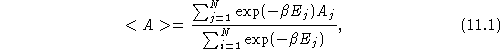where the sum passes over all states of the system, and where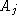is the value of A in the state j. To give this expression a quantum mechanical form, recall that to make a quantum observation we replace all observables A with their corresponding operators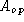. If one measures A for a system in state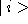, unlessis an eigenstate ofone gets different values for A in different measurements. If a large series of replicas of a given system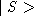are examined, the quantum average value of A is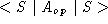. Applying these principles to eq. 11.1 replaces E and A with their operator forms, so for a quantum system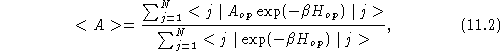the sum on j passing over all states of the system.

Equation 11.2 is significant because it embodies a double average, one average being quantum mechanical and the other statistical mechanical:

1) The quantum averages are indicated by the terms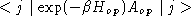and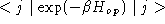. The first term gives the expectation value of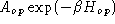in the state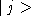, while the second term gives the expectation value of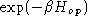in the same state. If the stateswere eigenstates ofand, the quantum average would have a particularly simple form, namely eq. 11.1. If thewere not eigenstates ofand, taking the expectation values would require expanding thein appropriate eigenstates, as shown in eqs. 10-31 and 10-32.

2) The statistico-mechanical average is indicated by the sums on j, which reflect averages over all states of the system, the various terms being given their canonical statistical weights.

Throughout the treatment of quantum systems, all ensemble averages will have this double nature. In each case, there will be a thermal average over different states of the system. Within each term of the thermal average, there will be a quantum average to obtain the expectation values ofand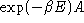within that state. The sequence of taking the averages is significant, the quantum average being taken separately for each state, the various quantum averages then being combined to yield a thermal average. This order of taking the two averages is consistent with the thus-far established image of an ensemble average. To averagewithin the classical canonical ensemble, the function A is evaluated separately in each element of the ensemble, a thermally weighted average of A from different states of the ensemble then being taken. In the quantum canonical ensemble, evaluatingin each element of the ensemble requires computing its expectation value. After the expectation value has been evaluated separately for each element of the quantum ensemble, the thermally weighted statistico-mechanical average ofis taken, as in the non-quantum case.

In Lectures 8 and 9, statistical mechanics was applied to quantized systems: the rigid rotor, the quantized-spin in an external field, and the harmonic oscillator. The partition function was written as a sum over the energy eigenstates of the system. Energies computed quantum-mechanically, and a discrete form of eq. 11.1, then gave values for Q, <E>, and other quantities.

A first question is whether or not we were justified in using the list of energy eigenstates of the system as a correct list of all states of the system. There are variables which do not commute with. If we had written the sum of eq. 11.1 while using some other set of eigenstates, instead of using energy eigenstates, would we have obtained the same value for Q? If we get different values for Q when we use different sets of basis vectors, how d0 we know which eigenstates we should use to take an average over the canonical ensemble? Fortunately, it turns out that the choice of eigenstates is irrelevant. The termsandcan be viewed as matrix elements of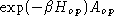and. The sums over states of eq. 11.1 are traces of matrices. The trace of a matrix is invariant to the choice of basis vectors. While, e. g. ,changes when the basis vectorsare changed, the sums in eq. 11.1 are independent of the choice of basis vectors. Any set of basis vectors which is a complete orthogonal set of states is equally acceptable for evaluating Q or <A>, because eq. 11.2 gives the same value for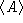regardless of the choice of basis vector.

The independence of ensemble averages and partition functions from the choice of basis vectors has practical applications in statistical mechanics. To obtain the thermodynamic properties of a quantum system, one only needs to evaluate its partition function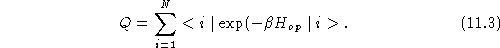Q is easily evaluated if theare energy eigenstates, because in that case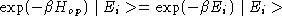andSuppose one has a system for which a set of basis vectors (a ``complete set of states'') have been found, but those basis states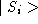are not the energy eigenstates. Q can be computed from eq. 11.3 while using the basis vectorsas the states of the system. The individual matrix elements will be more tedious to evaluate, because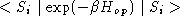involve the quantum average ofover the mixed (with respect to energy eigenstates) state. However, use of therather than the energy eigenstates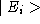will not affect the sum Q of the diagonal matrix elements of.

As a demonstration of the independence of Q and the choice of basis vectors, consider the isolated spin system of Lecture 8. For a single spin the energy was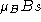for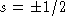, the partition function being written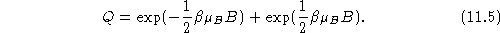This Q may be rewritten as a double quantum and thermal average, using the notation of the previous Lecture. Eq. 11.5 isforand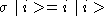. Eqs. 11.5 and 11.6 are written in terms of energy eigenstates. An alternative to energy eigenstates are the helicity eigenstates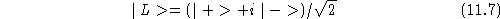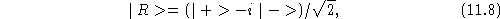in terms of which the partition function is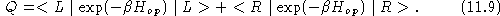It may be confirmed by direct computation that Q from eq. 11.9 agrees with Q in eq. 11.5.

A technical issue arises in the quantum evaluation of ensemble averages. There is no physical guarantee that A andcommute, in which caseand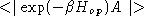need not be equal. This issue arises already in conventional quantum mechanics, in the evaluation of such averages as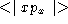. The orthodox resolution for calculating the expectation value of non-commuting variables is to evaluate the symmetrized product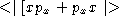. [When I last investigted the question, no one had proposed an experiment to test the orthodox resolution.]Next: Replacement of Sums over All Up: Chapter 11 Previous: Chapter 11

Nicholas V Sushkin
Sun Jun 30 15:55:07 EDT 1996